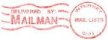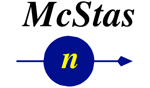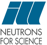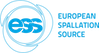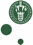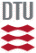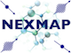McStas: Lens Component

# The `Lens` Component

Refractive lens with absorption, incoherent scattering and surface imperfection.

## Identification

• Author: C. Monzat/E. Farhi/S. Desert/G. Euzen
• Origin: ILL/LLB
• Date: 2009
• Version:(Unknown)

## Description

```Refractive Lens with absorption, incoherent scattering and surface imperfection.
Geometry may be:
spherical (use r1 and r2 to specify radius of curvature),
planar    (use phiy1 and phiy2 to specify rotation angle of plane w.r.t beam)
parabolic (use focus1 and focus2 as optical focal length).

Optionally, you can specify the 'geometry' parameter as a OFF/PLY file name.
The complex geometry option handles any closed non-convex polyhedra.
It computes the intersection points of the neutron ray with the object
transparently, so that it can be used like a regular sample object.
It supports the PLY, OFF and NOFF file format but not COFF (colored faces).
Such files may be generated from XYZ data using:
qhull < coordinates.xyz Qx Qv Tv o > geomview.off
or
powercrust coordinates.xyz
and viewed with geomview or java -jar jroff.jar (see below).

The lens cross-section is seen as a 2*radius disk from the beam Z axis, except
when a 'geometry' file is given.
Usually, you should stack more than one of these to get a significant effect
on the neutron beam, so-called 'compound refractive lens'.

The focal length for N lenses with focal 'f' is f/N, where f=R/(1-n)
and R = r/2   for a spherical lens with curvature radius 'r'
R = focus for a parabolic lens with focal of the parabola
and n = sqrt(1-(lambda*lambda*rho*bc/PI)) with
bc  = sqrt(fabs(sigma_coh)*100/4/PI)*1e-5
rho = density*6.02214179*1e23*1e-24/weight

Common materials: Should have high coherent, and low incoherent and absorption cross sections
Be:            density=1.85,  weight=9.0121, sigma_coh=7.63,  sigma_inc=0.0018,sigma_abs=0.0076
Pb:            density=11.115,weight=207.2,  sigma_coh=11.115,sigma_inc=0.003, sigma_abs=0.171
Pb206:                                     sigma_coh=10.68, sigma_inc=0    , sigma_abs=0.03
Pb208:                                     sigma_coh=11.34, sigma_inc=0    , sigma_abs=0.00048
Zr:            density=6.52,  weight=91.224, sigma_coh=6.44,  sigma_inc=0.02,  sigma_abs=0.185
Zr90:                                      sigma_coh=5.1,   sigma_inc=0    , sigma_abs=0.011
Zr94:                                      sigma_coh=8.4,   sigma_inc=0    , sigma_abs=0.05
Bi:            density=9.78,  weight=208.98, sigma_coh=9.148, sigma_inc=0.0084,sigma_abs=0.0338
Mg:            density=1.738, weight=24.3,   sigma_coh=3.631, sigma_inc=0.08,  sigma_abs=0.063
MgF2:          density=3.148, weight=62.3018,sigma_coh=11.74, sigma_inc=0.0816,sigma_abs=0.0822
diamond:       density=3.52,  weight=12.01,  sigma_coh=5.551, sigma_inc=0.001, sigma_abs=0.0035
Quartz/silica: density=2.53,  weight=60.08,  sigma_coh=10.625,sigma_inc=0.0056,sigma_abs=0.1714
Si:            density=2.329, weight=28.0855,sigma_coh=2.1633,sigma_inc=0.004, sigma_abs=0.171
Al:            density=2.7,   weight=26.98,  sigma_coh=1.495, sigma_inc=0.0082,sigma_abs=0.231
perfluoropolymer(PTFE/Teflon/CF2):
density=2.2,   weight=50.007, sigma_coh=13.584,sigma_inc=0.0026,sigma_abs=0.0227
Organic molecules with C,O,H,F

%BUGS
parabolic shape is not fully validated yet, but should do still...

```
WARNING: This is a contributed Component.

## Input parameters

Parameters in boldface are required; the others are optional.
Name Unit Description Default
r1 m radius of the first circle describing the lens. r>0 means concave face, r<0 means convex, r=0 means plane 0
r2 m radius of the second circle describing the lens r>0 means concave face, r<0 means convex, r=0 means plane 0
focus1 m focal of the first parabola describing the lens 0
focus2 m focal of the second parabola describing the lens 0
phiy1 deg angle of plane1 (r1=0) around y vertical axis 0
phiy2 deg angle of plane2 (r2=0) around y vertical axis 0
thickness m thickness of the lens between its two surfaces 0.001
sigma_coh barn coherent cross section 11.74
sigma_inc barn incoherent cross section 0.0816
sigma_abs barn thermal absorption cross section 0.0822
density g/cm3 density of the material for the lens 3.148
weight g/mol molar mass of the material 62.3018
p_interact 1 MC Probability for scattering the ray; otherwise transmit 0.1
focus_aw deg vertical angle to forward focus after scattering 10
focus_ah deg horizontal angle to forward focus after scattering 10
RMS Angs root mean square roughness of the surface 0
geometry str Name of an Object File Format (OFF) or PLY file for complex geometry. The OFF/PLY file may be generated from XYZ coordinates using qhull/powercrust 0

## Output parameters

Name Unit Description Default
rho
bc
my_s
my_a_v
mean_n
events

• Source code for `Lens.comp`.
• M. L. Goldberger et al, Phys. Rev. 71, 294 - 310 (1947)
• Sears V.F. Neutron optics. An introduction to the theory of neutron optical phenomena and their applications. Oxford University Press, 1989.
• H. Park et al. Measured operationnal neutron energies of compound refractive lenses. Nuclear Instruments and Methods B, 251:507-511, 2006.
• J. Feinstein and R. H. Pantell. Characteristics of the thick, compound refractive lens. Applied Optics, 42 No. 4:719-723, 2001.
• Geomview and Object File Format (OFF)
• Java version of Geomview (display only) jroff.jar
• qhull
• powercrust

Generated automatically by McDoc, Peter Willendrup <peter.willendrup@risoe.dk> / Tue May 26 13:03:59 2020